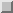Null ProcessesNext: Nonblocking Communication Up: Point-to-Point Communication Previous: Send-Receive

# Null Processes

null process   In many instances, it is convenient to specify a ``dummy'' source or destination for communication.

In the Jacobi example, this will avoid special handling of boundary processes. This also simplifies handling of boundaries in the case of a non-circular shift, when used in conjunction with the functions described in Chapter.

The special value MPI_PROC_NULL can be used MPI_PROC_NULL instead of a rank wherever a source or a destination argument is required in a communication function. A communication with process MPI_PROC_NULL has no effect. A send to MPI_PROC_NULL succeeds and returns as soon as possible. A receive from MPI_PROC_NULL succeeds and returns as soon as possible with no modifications to the receive buffer. When a receive with source = MPI_PROC_NULL is executed then the status object returns source = MPI_PROC_NULL, tag = MPI_ANY_TAG and count = 0.

We take advantage of null processes to further simplify the parallel Jacobi code. Jacobi, with null processes

```Example2.15 Jacobi code -  version of parallel code using sendrevc and null
processes.
...
REAL, ALLOCATABLE A(:,:), B(:,:)
...
! Compute number of processes and myrank
CALL MPI_COMM_SIZE(comm, p, ierr)
CALL MPI_COMM_RANK(comm, myrank, ierr)

! compute size of local block
m = n/p
IF (myrank.LT.(n-p*m)) THEN
m = m+1
END IF

! Compute neighbors
IF (myrank.EQ.0) THEN
left = MPI_PROC_NULL
ELSE
left = myrank - 1
END IF
IF (myrank.EQ.p-1)THEN
right = MPI_PROC_NULL
ELSE
right = myrank+1
END IF

! Allocate local arrays
ALLOCATE (A(0:n+1,0:m+1), B(n,m))
...
!Main Loop
DO WHILE(.NOT.converged)
! compute
DO j=1, m
DO i=1, n
B(i,j)=0.25*(A(i-1,j)+A(i+1,j)+A(i,j-1)+A(i,j+1))
END DO
END DO

DO j=1, m
DO i=1, n
A(i,j) = B(i,j)
END DO
END DO

! Communicate
CALL MPI_SENDRECV(B(1,1),n, MPI_REAL, left, tag,
(A(1,0),n, MPI_REAL, left, tag, comm,
status, ierr)
CALL MPI_SENDRECV(B(1,m),n, MPI_REAL, right, tag,
(A(1,m+1),n, MPI_REAL, right, tag, comm,
status, ierr)
END IF
...

```

Jack Dongarra
Fri Sep 1 06:16:55 EDT 1995